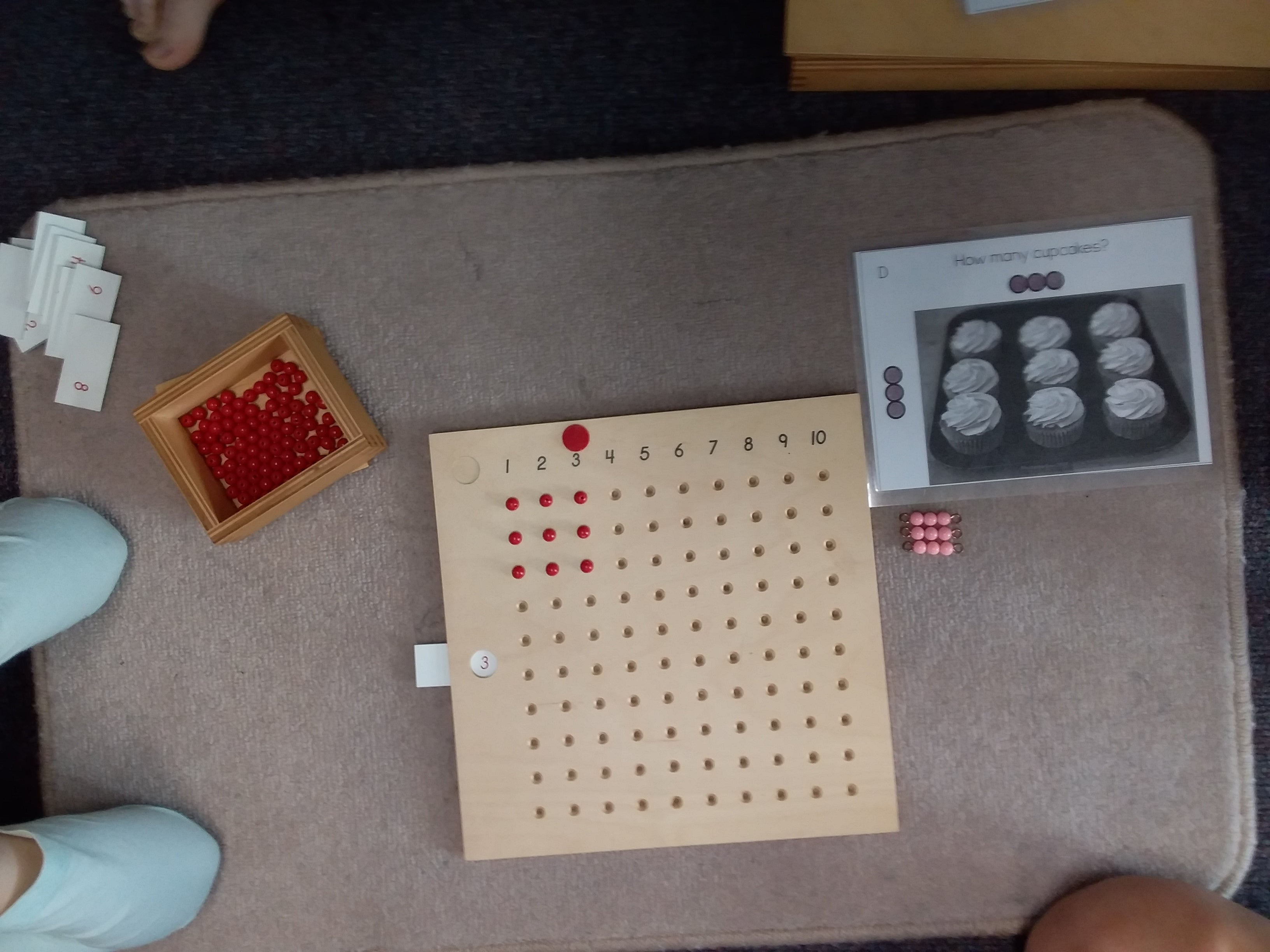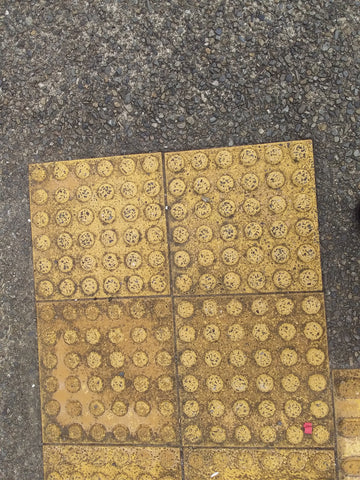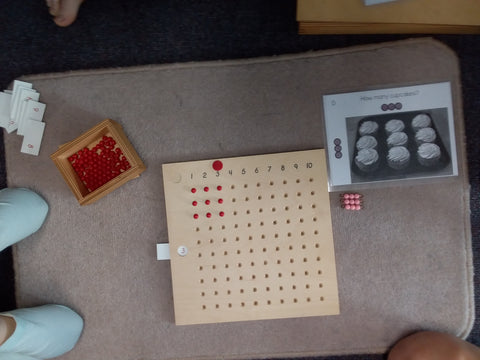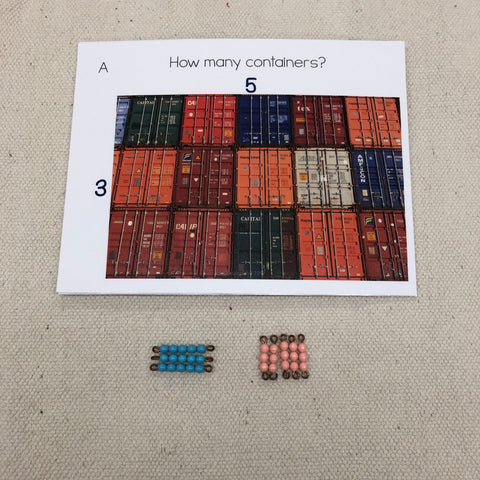# Moving from Addition to Multiplication with Arrays

Have you heard of arrays? I must admit that before upskilling in my mainstream maths knowledge I hadn't! You are probably familiar with them though

Let's take this piece of footpath.Each individual square has 6 dots across and 6 dots down.

A student may count this a number of ways

A) 1:1 pointing and counting to every dot

B) skip counting  6 + 6 + 6 + 6

C) Using doubles and then adding doubles onto doubles eg. (6 + 6) + (6+6) + (6+6)

D) Using multiplication facts 6 x 6

Today I am going to concentrate on a lesson I did with B, C and D from the list above. The students I was working with are able to skip count, some of them know their doubles however they are at the start of their knowledge in multiplication and as a group they tend to revert to simple methods.

We had had a lesson on the multiplication bead board last week however things didn't seem to make much of an impression so I decided to go back to arrays.

I showed the students various pictures like these below and encouraged them to count the objects. We then discussed the strategies and talked about what was most efficient.

One of the best bits was when we had a mathematical argument. One child decided just to count the objects around the edge. Another child in the group said 'no' and then showed the first child that you could make groups with the columns to count. Another child counted in 2s to work out the amount. We finally worked out that skip counting was probably most efficient however knowing our multiplication facts would make it even faster. I showed the students how the multiplication board could help us with this and also showed them what various arrays looked like with the colored bead bars.Here are some other examples of cards in the array pack I made and used. They are available hereThe child could count the cookies in rows (4 + 4 + 4 + 4 + 4 + 4) or in rows

(6 + 6+ 6 + 6). It is good if you can make a link to the relevant multiplication equation.I appreciate the materials that go well with the actual Montessori apparatus.

Shamim

This is great! Thank you for sharing! Is it possible that a child moves on to multiplication before subtraction?

Grace

Nice supplementary exercise .

Shamim